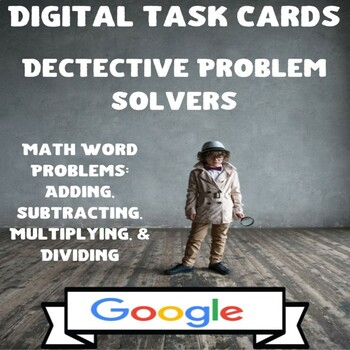# Digital Task Cards-Problem Solving Detectives: Math Word ProblemsSubject
Resource Type
File Type

PDF

(3 MB)
Product Rating
Standards
Also included in:
1. Use these no prep CCSS aligned, self-checking, interactive digital task cards on Google Slides as a highly engaging assessment, exit ticket, math center, or just interactive additional practice.What a great way to check for understanding while students have a blast!!! These Digital Task Cards-Proble
\$7.50
\$4.20
Save \$3.30
2. There is no better way to prepare for upcoming standardized testing like I-Learn than implementing the products in this bundle. Included in this Math Test Prep BUNDLE: Word Problems are (5) Digital Escape Rooms on Google Forms, (3) sets of Interactive Digital Task Cards on Google Slides, and (6) se
\$32.50
\$23.40
Save \$9.10
• Product Description
• StandardsNEW

Use these CCSS aligned, self-checking, interactive digital task cards on Google Slides as a highly engaging assessment, exit ticket, math center, or just interactive additional practice.

What a great way to check for understanding while students have a blast!!! These Digital Task Cards-Problem Solving Detectives: Math Word Problems will be the easiest you have ever implemented as all you have to do is assign them in Google Classroom and students take it from there. Contained within the slides or 25 real-world math word problems, many of which are multi-step and multi-operation. A newly added feature to these interactive slides are funny and entertaining GIFs which are sure to be a crowd pleaser among students.

1) Specific directions on how students use the interactive digital task cards.

2) Specific directions with screen shots on how to save a copy of this to your Google Drive, which will also give you editing rights.

3) Specific directions with screen shots on how to assign them via Google Classroom.

5) A link to get a copy of the product.

With these Digital Task Cards-Problem Solving Detectives: Math Word Problems the directions are simple as correct responses force them to click to the next problem while incorrect responses force them to go back and re-work the problem until they get it correct.

Find the area of a rectangle with fractional side lengths by tiling it with unit squares of the appropriate unit fraction side lengths, and show that the area is the same as would be found by multiplying the side lengths. Multiply fractional side lengths to find areas of rectangles, and represent fraction products as rectangular areas.
Apply and extend previous understandings of multiplication to multiply a fraction or whole number by a fraction.
Solve word problems involving addition and subtraction of fractions referring to the same whole, including cases of unlike denominators, e.g., by using visual fraction models or equations to represent the problem. Use benchmark fractions and number sense of fractions to estimate mentally and assess the reasonableness of answers. For example, recognize an incorrect result 2/5 + 1/2 = 3/7, by observing that 3/7 < 1/2.
Add and subtract fractions with unlike denominators (including mixed numbers) by replacing given fractions with equivalent fractions in such a way as to produce an equivalent sum or difference of fractions with like denominators. For example, 2/3 + 5/4 = 8/12 + 15/12 = 23/12. (In general, 𝘢/𝘣 + 𝘤/𝘥 = (𝘢𝘥 + 𝘣𝘤)/𝘣𝘥.)
Solve word problems involving multiplication of a fraction by a whole number, e.g., by using visual fraction models and equations to represent the problem. For example, if each person at a party will eat 3/8 of a pound of roast beef, and there will be 5 people at the party, how many pounds of roast beef will be needed? Between what two whole numbers does your answer lie?
Total Pages
N/A
Included
Teaching Duration
N/A
Report this Resource to TpT
Reported resources will be reviewed by our team. Report this resource to let us know if this resource violates TpT’s content guidelines.\$2.00
List Price:
\$2.50
You Save:
\$0.50